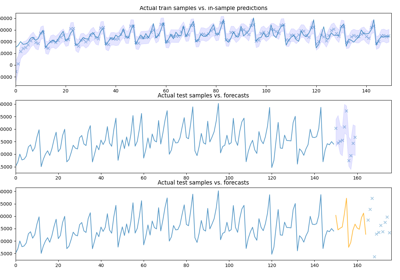# pmdarima.preprocessing.FourierFeaturizer¶

class pmdarima.preprocessing.FourierFeaturizer(m, k=None, prefix=None)[source][source]

Fourier terms for modeling seasonality

This transformer creates an exogenous matrix containing terms from a Fourier series, up to order k. It is based on R::forecast code . In practice, it permits us to fit a seasonal time series without seasonal order (i.e., seasonal=False) by supplying decomposed seasonal Fourier terms as an exogenous array.

The advantages of this technique, per Hyndman :

• It allows any length seasonality
• The seasonal pattern is smooth for small values of K (but more wiggly seasonality can be handled by increasing K)
• The short-term dynamics are easily handled with a simple ARMA error

The disadvantage is that the seasonal periodicity of the time series is assumed to be fixed.

Functionally, this is a featurizer. This means that exogenous features are derived from y, as opposed to transforming an existing exog array. It also behaves slightly differently in the transform() stage than most other exogenous transformers in that exog is not a required arg, and it takes **kwargs. See the transform() docstr for more info.

Parameters: m : int The seasonal periodicity of the endogenous vector, y. k : int, optional (default=None) The number of sine and cosine terms (each) to include. I.e., if k is 2, 4 new features will be generated. k must not exceed m/2, which is the default value if not set. The value of k can be selected by minimizing the AIC. prefix : str or None, optional (default=None) The feature prefix

Notes

• Helpful for long seasonal periods (large m) where seasonal=True seems to take a very long time to fit a model.

References

Examples

>>> import pandas as pd
>>> from pmdarima.preprocessing import FourierFeaturizer
>>> trans = FourierFeaturizer(12, 4)
>>> y_prime, exog = trans.fit_transform(y)
FOURIER_S12-0     FOURIER_C12-0    ...     FOURIER_S12-3  FOURIER_C12-3
0       0.500000      8.660254e-01    ...      8.660254e-01           -0.5
1       0.866025      5.000000e-01    ...     -8.660255e-01           -0.5
2       1.000000     -4.371139e-08    ...      1.748456e-07            1.0
3       0.866025     -5.000001e-01    ...      8.660253e-01           -0.5
4       0.500000     -8.660254e-01    ...     -8.660255e-01           -0.5


Methods

 fit(y[, exogenous]) Fit the transformer fit_transform(y[, exogenous]) Fit and transform the arrays get_params([deep]) Get parameters for this estimator. set_params(**params) Set the parameters of this estimator. transform(y[, exogenous, n_periods]) Create Fourier term features update_and_transform(y, exogenous, **kwargs) Update the params and return the transformed arrays
__init__(m, k=None, prefix=None)[source][source]

Initialize self. See help(type(self)) for accurate signature.

fit(y, exogenous=None)[source][source]

Fit the transformer

Computes the periods of all the Fourier terms. The values of y are not actually used; only the periodicity is used when computing Fourier terms.

Parameters: y : array-like or None, shape=(n_samples,) The endogenous (time-series) array. exogenous : array-like or None, shape=(n_samples, n_features), optional The exogenous array of additional covariates. If specified, the Fourier terms will be column-bound on the right side of the matrix. Otherwise, the Fourier terms will be returned as the new exogenous array.
fit_transform(y, exogenous=None, **transform_kwargs)[source]

Fit and transform the arrays

Parameters: y : array-like or None, shape=(n_samples,) The endogenous (time-series) array. exogenous : array-like or None, shape=(n_samples, n_features), optional The exogenous array of additional covariates. **transform_kwargs : keyword args Keyword arguments required by the transform function.
get_params(deep=True)[source]

Get parameters for this estimator.

Parameters: deep : bool, default=True If True, will return the parameters for this estimator and contained subobjects that are estimators. params : mapping of string to any Parameter names mapped to their values.
set_params(**params)[source]

Set the parameters of this estimator.

The method works on simple estimators as well as on nested objects (such as pipelines). The latter have parameters of the form <component>__<parameter> so that it’s possible to update each component of a nested object.

Parameters: **params : dict Estimator parameters. self : object Estimator instance.
transform(y, exogenous=None, n_periods=0, **_)[source][source]

Create Fourier term features

When an ARIMA is fit with an exogenous array, it must be forecasted with one also. Since at predict time in a pipeline we won’t have y (and we may not yet have an exog array), we have to know how far into the future for which to compute Fourier terms (hence n_periods).

This method will compute the Fourier features for a given frequency and k term. Note that the y values are not used to compute these, so this does not pose a risk of data leakage.

Parameters: y : array-like or None, shape=(n_samples,) The endogenous (time-series) array. This is unused and technically optional for the Fourier terms, since it uses the pre-computed n to calculate the seasonal Fourier terms. exogenous : array-like or None, shape=(n_samples, n_features), optional The exogenous array of additional covariates. If specified, the Fourier terms will be column-bound on the right side of the matrix. Otherwise, the Fourier terms will be returned as the new exogenous array. n_periods : int, optional (default=0) The number of periods in the future to forecast. If n_periods is 0, will compute the Fourier features for the training set. n_periods corresponds to the number of samples that will be returned.
update_and_transform(y, exogenous, **kwargs)[source][source]

Update the params and return the transformed arrays

Since no parameters really get updated in the Fourier featurizer, all we do is compose forecasts for n_periods=len(y) and then update n_.

Parameters: y : array-like or None, shape=(n_samples,) The endogenous (time-series) array. exogenous : array-like or None, shape=(n_samples, n_features) The exogenous array of additional covariates. **kwargs : keyword args Keyword arguments required by the transform function.

## Examples using pmdarima.preprocessing.FourierFeaturizer¶Pipelines with auto_arima